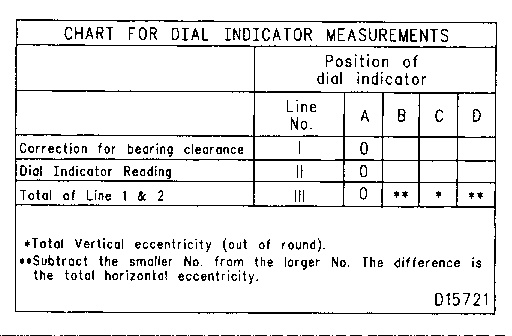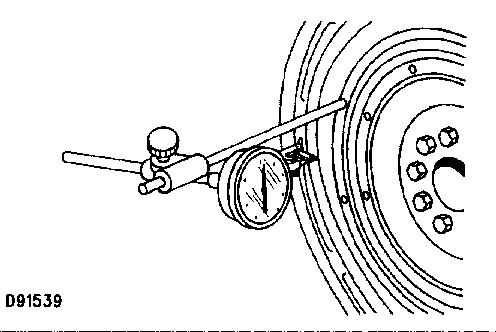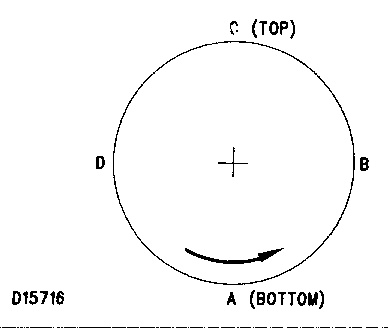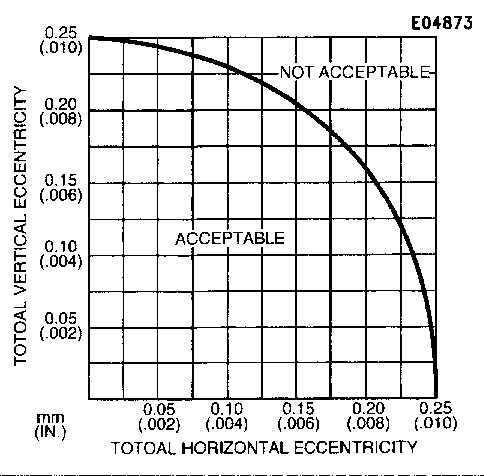# 3208 – Flywheel Housing BoreNOTE: Write the dial indicator measurements with their positive (+) and negative (-) notation (signs). This rotation is necessary for making the calculations in the chart correctly.1. With the dial indicator in position at (C), adjust the dial indicator to “0” (zero). Push the crankshaft up against the top bearing. Write the measurement for bearing clearance on line 1 in column (C).

2. Divide the measurement from Step 1 by 2. Write this number on line 1 in columns (B) & (D).3. Turn the crankshaft to put the dial indicator at (A). Adjust the dial indicator to “0” (zero).

4. Turn the crankshaft COUNTERCLOCKWISE to put the dial indicator at (B). Write the measurement in the chart.

5. Turn the crankshaft COUNTERCLOCKWISE to put the dial indicator at (C). Write the measurement in the chart.

6. Turn the crankshaft COUNTERCLOCKWISE to put the dial indicator at (D). Write the measurement in the chart.

7. Add lines I and II by columns.

8. Subtract the smaller number from the larger number in line III in columns (B) & (D). The result is the horizontal “eccentricity” (out of round). Line III, column (C) is the vertical eccentricity.

9. On the graph for total eccentricity find the point of intersection of the lines for vertical eccentricity and horizontal eccentricity.

10. If the point of intersection is in the range marked “Acceptable” the bore is in alignment. If the point of intersection is in the range marked “Not Acceptable” the flywheel housing must be changed.Total Horizontal Eccentricity